×

Search anything:

# Different Basic Operations in CNN

#### Machine Learning (ML)Get this book -> Problems on Array: For Interviews and Competitive Programming

Convolutional Neural Network is the type of neural network which is used for analysis of visual inputs. Some popular applications of CNN includes image classification, image recognition, Natural language processing etc.
Now, there are certain steps/operations that are involved CNN. These can be categorized as follows:

1. Convolution operation
2. Pooling
3. Flattening
5. Fully connected layers
6. Activation function (like Softmax)
7. Batch Normalization

etc. Let us explore them individually.

# i. CONVOLUTION OPERATION

I. PURPOSE
Convolution operations is the first and one of the most important step in the functioning of a CNN. Convolution operation focuses on extracting/preserving important features from the input (image etc).
To understand this operation, let us consider image as input to our CNN. Now when image is given as input, they are in the form of matrices of pixels. If the image is grayscale, then the image is considered as a matrix, each value in the matrix ranges from 0-255. We can even normalize these values lets say in range 0-1. 0 represents white and 1 represents black. If the image is colored, then three matrices representing the RGB colors with each value in range 0-255. The same can be seen in the images below: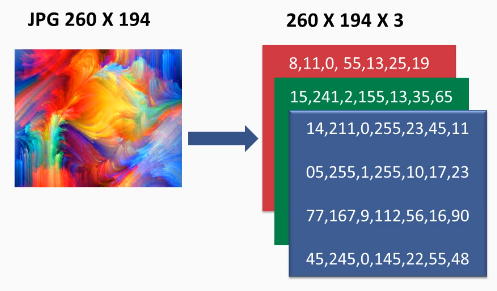Fig 1: Colored image matrices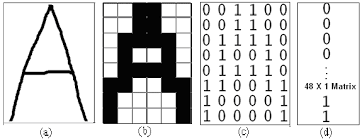Fig 2: Grascale image matrix

II. MATHEMATICAL OPERATION
Coming to convolution operation, let us consider an input image. Now for convolution operation, filters or kernels are used. The following mathematical operation is performed:
Let size of image: NxN
Let size of filer: FxF
Then (NxN)*(FxF)= (N-F+1)x(N-F+1)
*= Convolution operation
All these kernels, input channels etc are the hyper parameters. The result of each layer is passed on to the next one.

III. EXAMPLE
Consider the image given below: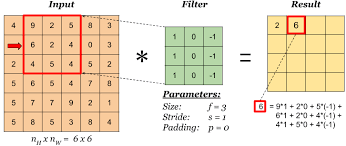Here, input of size 6x6 is given and kernel of size 3x3 is used. The feature map obtained in of size 4x4.
To increase non-linearity in the image, Rectifier function can be applied to the feature map.
Finally, after the convolution step is completed and feature map is obtained, this map is given as input to the pooling layer.

# ii. POOLING

This is another important step in convolutional neural network.
I. PURPOSE
This step helps to maintain spatial invariance or even deal with other kind of distortions in the process. This means that if we are trying to perform image recognition or something like checking if the image contains a dog, there is a possibility that the image might not be straight (maybe tilted), or texture difference is there, the object size in the image is small etc. So, this should not let our model to provide incorrect output. This is what pooling is all about. There can be different types of pooling like min pooling, max pooling etc. It helps to preserve the essential features. What we obtain is called pooled feature map. Here the size is reduced, features are preserved and distortions are dealt with.

II. MATHEMATICAL OPERATION
Let us consider dimensions of feature maps be HxWxC.
Let H- height of feature map
W- width of feature map
C- number of channels in the feature map
F- filter and S- stride length
Then the output image will be:
[(H-F)/S] +1 x [(W-F)/S]+1 x C

III. TYPES OF POOLING
Mentioned below are some types if pooling that are used:
1. Max Pooling: In max pooling, the maximum value is taken from the group of values of patch feature map.
2. Minimum Pooing: In this type of pooling, the minimum value is taken from the patch in feature map.
3. Average Pooling: Here, the average of values is taken.
4. Adaptive Pooling: In this type of pooling, we only need to define the output size we need for the feature map. Parameters such as stride etc are automatically calculated
.

IV. EXAMPLE
Let is take an example to understand pooling better: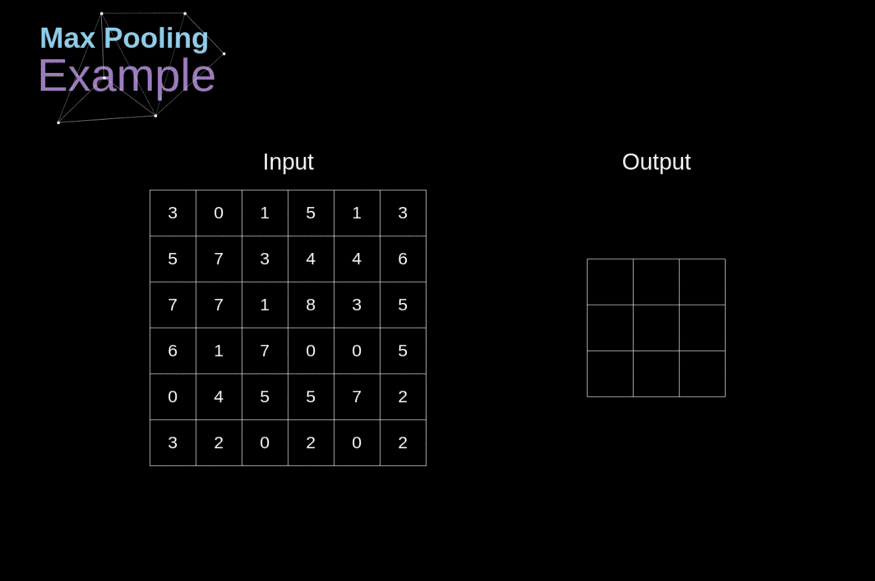In the above image of size 6x6, we can see that on the feature map, max pooling is applied with stride 2 and filter 2 or 2x2 window. This operation reduces the size of the data and preserves the most essential features.
The output obtained after the pooling layer is called the pooled feature map.

I. PURPOSE
CNN has offered a lot of promising results but there are some issues that comes while applying convolution layers. There are two significant problems:

1. When we apply convolution operation, based on the size of image and filter, the size of the resultant processed image reduces according to the following rule:
Let image size: nxn
Let filer size: mxm
Then, resultant image size: (n-m+1)x(n-m+1)
2. Another problem comes with the pixel values in the edges of the image matrix. The values on the edges of the matrix do not contribute as much as the values in the middle. Like if some pixel value resides in the corner i.e. (0,0) position, then it will be considered only once in the convolution operation, while values in the middle will be considered multiple times.
To overcome these important problems, padding is the solution. In padding, we layer of values like adding layer(s) of zeros around the feature matrix, as shown in the below image.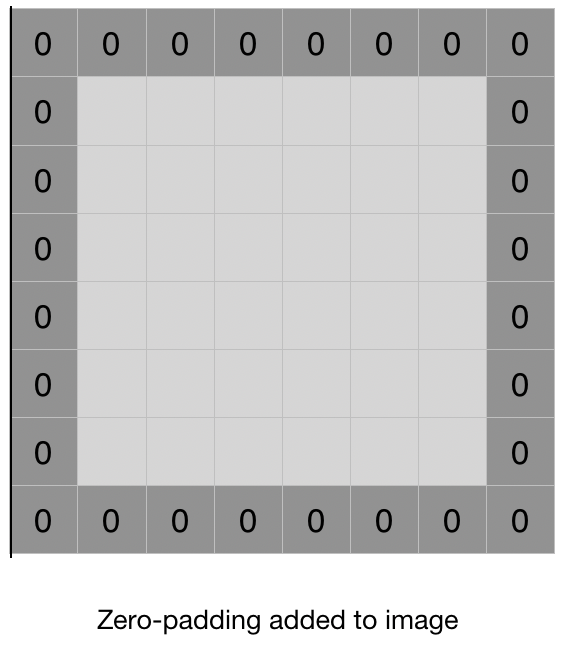II. MATHEMATICAL OPERATION
Now let us take the same example where image is of size (nxn) and filter of size (mxm). If we add p layers of padding, then the resultant image will be of size (n+2p-m+1)x(n+2p-m+1).

III. EXAMPLE
If we take an image of size 9x9 and filer of size 3x3, if we add 1 layer of padding, then the image after applying convolution operation if of size 9x9. Hence the problem of reduced size of image after convolution is taken care of and because of padding, the pixel values on the edges are now somewhat shifted towards the middle.

# iv. Flattening

This is a step that is used in CNN but not always. Based on the upcoming layers in the CNN, this step is involved.
What happens here is that the pooled feature map (i.e. the matrix) is converted into a vector. And this vector plays the role of input layer in the upcoming neural networks.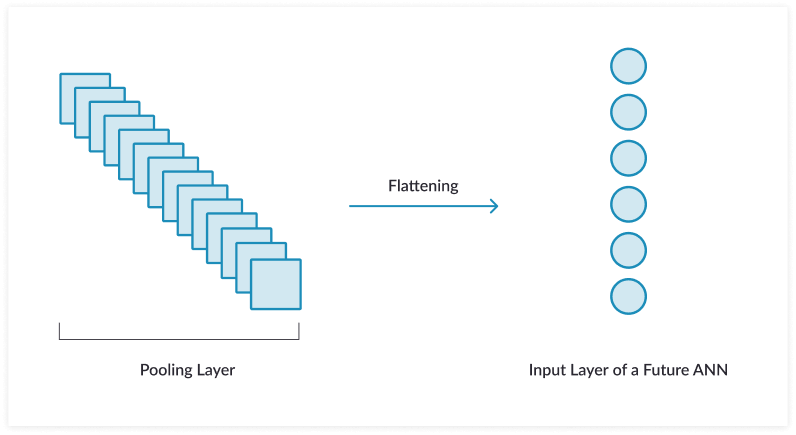# v. Fully connected layers

After several layers of convolution and pooling operations are completed, now the final output is given to the fully connected layer. This is basically a neural network in which each neuron is connected to every other neuron in the previous layer.
All the recognition and classification parts are done by this neural network.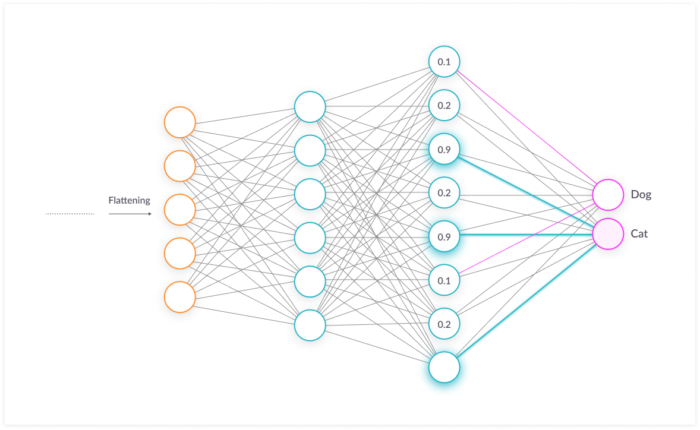In the above image, the output after flattening is given to the fully connected layer and this network helps is in classifying the image as either cat or dog.

# Activation function

Activation function plays very important role. There are many activation functions present like Linear, polynomial etc. But in CNN, one of the most popular activation function used is the RELU function.
To know more about activation functions and types, checkout the links in references.

# SOFTMAX

Softmax activation function has very useful when it comes to classification problems. Let us consider the case of image classification. What is does is that for each class, it will generate the probabilities i.e. if there are n classes, then for a given input image, it will generate probabilities for each of the n classes and the one with the highest probability is the output.
The following is the formula for SoftMax function: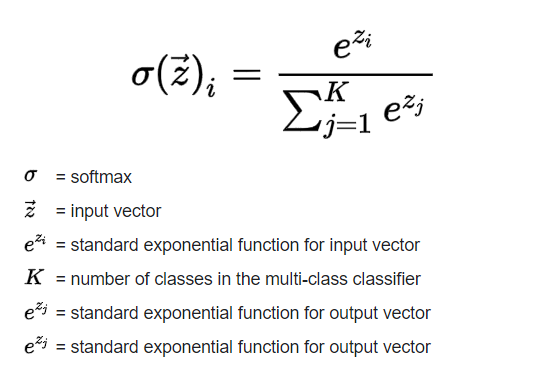# Batch Normalization

Sometimes, one additional step called batch normalization is applied after certain layers in the CNN. Batch normalization is a technique used to increase the stability of a neural network. It helps our neural network to work with better speed and provide more efficient results. Let us say we have different parameters and the values of those parameters differ on a great scale i.e. if parameter A has value in range 1-10 and parameter B has values in range 1-million, then some issues might occur. This is where batch norm will help. It can help us by normalizing values in a certain range (lets say 0-1). Now all the values are changed and ranges b/w 0 and 1.
For more details, please find the link to article on batch normalization in references.

Now Let is explore one of the most popular CNN model GoogleNet.

GoogleNet is a deep and complex CNN model. The overall architecture includes 22 layers (27layers if pooling layers are included). The following diagram shows the layers: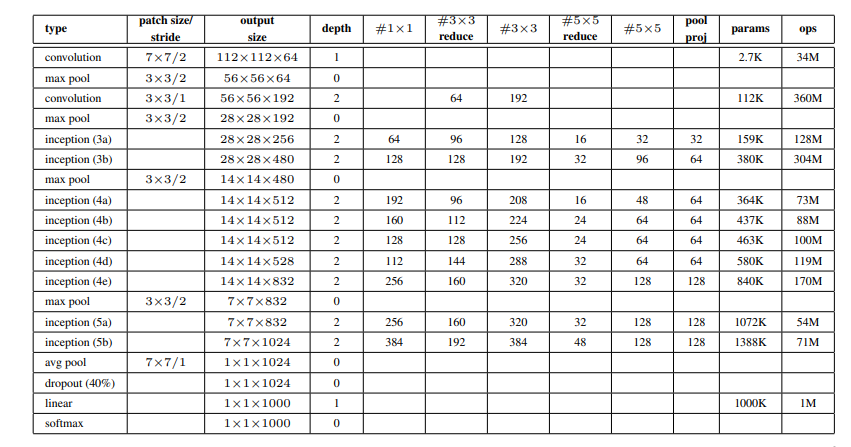It involves 9 inception modules. A basic inception module looks like this: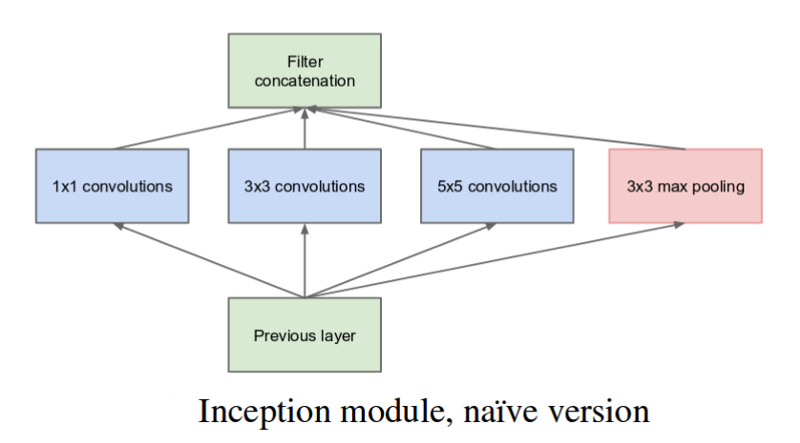Here variations of filters are present in parallel and finally the output is concatenated and sent to next layers.
GoogleNet has proven very efficient in many machine learning tasks such as Image classification, quantization, object detection, domain adaption, face recognition etc.

# CONCLUSION

In conclusion, we saw that steps that are involved in CNN are convolution, pooling, flattening and fully connected layers. Some addition steps are also involved like batch normalization, addition layers for activation function etc.
There can be several layers of each operation. The more complex the network, the better accuracy may be obtained.

1. Batch Normalization: https://iq.opengenus.org/batch-normalization/
2. Activation Function: https://iq.opengenus.org/activation-functions-ml/
3. Types of Activation Functions: https://iq.opengenus.org/types-of-activation-function/#### Apoorva Kandpal

I am a Deep learning enthusiast and am a student at SRM Institute of Science & Technology. I am able to handle multiple tasks on a daily basis. I am always energetic and eager to learn new skills

Different Basic Operations in CNN